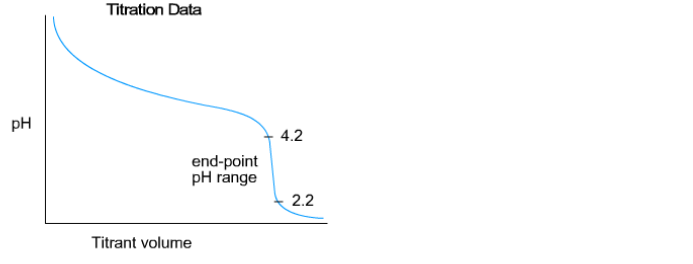# Problem: The titration shown here has an equivalence point at pH = 3.2. Identify which of the following indicators would be appropriate for this titration. Check all that apply.a. metacresol purple  pKa = 1.51, 8.32b. ethyl red  pKa = 5.42c. quinaldine red  pKa = 2.63d. bromothymol blue  pKa = 7.0 e. phenolphthalein  pKa = 9.50f. thymol blue  pKa = 1.65, 9.20

###### FREE Expert Solution
91% (173 ratings)
###### FREE Expert Solution
• If we will analyze the graph and the given info, we can see that  equivalence point is at 3.2

91% (173 ratings)###### Problem Details

The titration shown here has an equivalence point at pH = 3.2. Identify which of the following indicators would be appropriate for this titration. Check all that apply.

a. metacresol purple  pKa = 1.51, 8.32

b. ethyl red  pKa = 5.42

c. quinaldine red  pKa = 2.63

d. bromothymol blue  pKa = 7.0

e. phenolphthalein  pKa = 9.50

f. thymol blue  pKa = 1.65, 9.20What scientific concept do you need to know in order to solve this problem?

Our tutors have indicated that to solve this problem you will need to apply the Acid Base Indicators concept. If you need more Acid Base Indicators practice, you can also practice Acid Base Indicators practice problems.

What is the difficulty of this problem?

Our tutors rated the difficulty ofThe titration shown here has an equivalence point at pH = 3....as medium difficulty.

How long does this problem take to solve?

Our expert Chemistry tutor, Dasha took 2 minutes and 31 seconds to solve this problem. You can follow their steps in the video explanation above.

What professor is this problem relevant for?

Based on our data, we think this problem is relevant for Professor Thyagarajan's class at JHU.